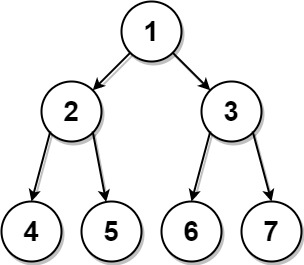889. Construct Binary Tree from Preorder and Postorder Traversal
Medium
2.4K
98

Given two integer arrays, `preorder` and `postorder` where `preorder` is the preorder traversal of a binary tree of distinct values and `postorder` is the postorder traversal of the same tree, reconstruct and return the binary tree.

If there exist multiple answers, you can return any of them.

Example 1:```Input: preorder = [1,2,4,5,3,6,7], postorder = [4,5,2,6,7,3,1]
Output: [1,2,3,4,5,6,7]
```

Example 2:

```Input: preorder = , postorder = 
Output: 
```

Constraints:

• `1 <= preorder.length <= 30`
• `1 <= preorder[i] <= preorder.length`
• All the values of `preorder` are unique.
• `postorder.length == preorder.length`
• `1 <= postorder[i] <= postorder.length`
• All the values of `postorder` are unique.
• It is guaranteed that `preorder` and `postorder` are the preorder traversal and postorder traversal of the same binary tree.
Accepted
87.5K
Submissions
123.3K
Acceptance Rate
71.0%

Seen this question in a real interview before?
1/4
Yes
No

Discussion (0)

Related Topics